# Fall sum or same

Find the probability that if you roll two dice, it will fall the sum of 10, or the same number will fall on both dice.

p =  0.2222

### Step-by-step explanation: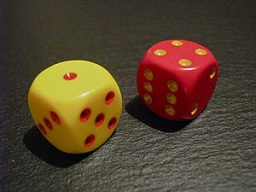Did you find an error or inaccuracy? Feel free to write us. Thank you!Tips to related online calculators
Would you like to compute count of combinations?

## Related math problems and questions:

• Dices throwsWhat is the probability that the two throws of the dice: a) Six falls even once b) Six will fall at least once
• We rollWe roll two dice A. - what is the probability that the sum of the falling numbers is at most 4 B. - is at least 10 C. - is divisible by 5?
• Sum or productWhat is the probability that two dice fall will have the sum 7 or product 12?
• ThrowWe throw 2 times with 2 dices. What is the probability that the first roll will fall more than sum of 9 and the second throw have sum 3 or does not have the sum 4?
• Two diceWe roll two dice. What is the probability that the sum of the falling numbers is greater than 3?
• Six on diceWhat is the probability that when throwing two dice will fall at least one six?
• DiceWe throw 10 times a playing dice. What is the probability that the six will fall exactly 4 times?
• Sum on diceWe have two dice. What is the greater likelihood of fall a total sum 7 or 8 ? (write 7, 8 or 0 if the probabilities are the same)?
• Three dice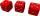What is the probability that when we roll three dice, we roll the numbers 1,2,3?
• DiceWe throw five times the dice. What is the probability that six fits exactly twice?
• Sum 10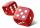What is the probability that two dice throw twice in a row will result the sum of 10?
• Roll the diceWhat is the probability that if we roll the dice, a number less than 5 falls?
• One dice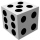Calculate the probability of one dice roll with the numbers 1, 2, 3, 4, 5, 6 on the walls. Write the results in a notebook in the shape of a fraction in the basic form: 2/3. a, The number 1 falls on the cube. b, The number 5 falls on the cube. c, An even
• Sum of fall dices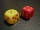What is the probability that the sum of 9 will fall on a roll of two dice? Hint: write down all the pairs that can occur as follows: 11 12 13 14 15. . 21 22 23 24. .. . 31 32. .. . . . . . .. . 66, count them, it's the variable n variable m: 36, 63,. .. .
• One threeWe throw two dice. What is the probability that max one three falls?
• Probability of intersectionThree students have a probability of 0.7,0.5 and 0.4 to graduated from university respectively. What is the probability that at least one of them will be graduated?
• Three colors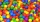Find the probability that 3 balls of the same color will be drawn from fate with 10 white, 10 red, and 10 blue balls.